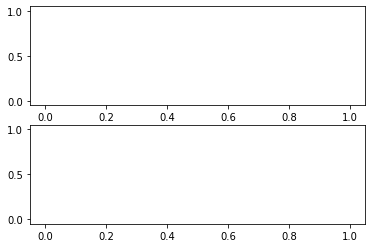:

import matplotlib.pyplot as plt
%matplotlib inline

:

from controlgame import ControlGame

:

game = ControlGame(runtime=30)  # seconds


# 52. Instructions¶

Run the cell below and click the “run” button. Then move the “MV” slider in a way which gets the controlled slider close to the setpoint. Your score increases more quickly when Controlled is near Setpoint. See how high your score can get by clicking run a couple of times. To see your performance graphed out, execute the next cell (game.plot())

:

game.ui()

:

game.plot():

import scipy.signal

:

ts = game.ts

:

G = scipy.signal.lti(2, [2, 0])

:

import numpy

:

LIMIT = 100

:

def score(ts, sps, cvs):
scores = 1 - numpy.minimum(numpy.abs(numpy.array(sps) - numpy.array(cvs)), LIMIT)/LIMIT

score = sum(scores)

return score

:

def sim(ts, mvs):
_, cvs, _ = scipy.signal.lsim(G, mvs, ts)

return cvs

:

def objective(mvs):
return -score(game.ts, game.sps, sim(game.ts, mvs))

:

objective(game.mvs)

---------------------------------------------------------------------------
IndexError                                Traceback (most recent call last)
----> 1 objective(game.mvs)

<ipython-input-13-96e53751337c> in objective(mvs)
1 def objective(mvs):
----> 2     return -score(game.ts, game.sps, sim(game.ts, mvs))

<ipython-input-12-d6eb717acf80> in sim(ts, mvs)
1 def sim(ts, mvs):
----> 2     _, cvs, _ = scipy.signal.lsim(G, mvs, ts)
3
4     return cvs

~/anaconda3/lib/python3.7/site-packages/scipy/signal/ltisys.py in lsim(system, U, T, X0, interp)
1944     xout = zeros((n_steps, n_states), sys.A.dtype)
1945
-> 1946     if T == 0:
1947         xout = X0
1948     elif T > 0:

IndexError: index 0 is out of bounds for axis 0 with size 0

[ ]:



[ ]:

import scipy.optimize

[ ]:

guesses = 1

[ ]:

bestmvs = game.mvs
for i in range(guesses):
sol = scipy.optimize.minimize(objective, bestmvs + 2*(numpy.random.rand(len(bestmvs))*2-1), bounds=[(-LIMIT, LIMIT)]*len(game.mvs))
print('Score:', -sol.fun)
bestmvs = sol.x
bestmvs[numpy.abs(bestmvs)<10] = 0

[ ]:

bestcvs = sim(ts, bestmvs)

[ ]:

fig, (axmv, axcv) = plt.subplots(2, 1)
axmv.plot(ts, bestmvs)
axcv.plot(ts, game.sps, ts, bestcvs)